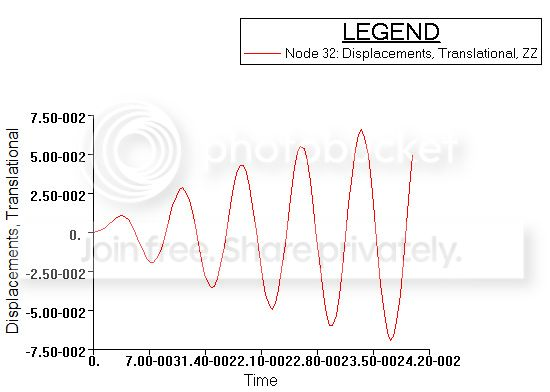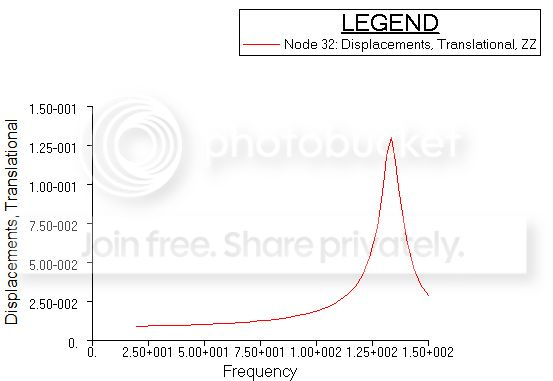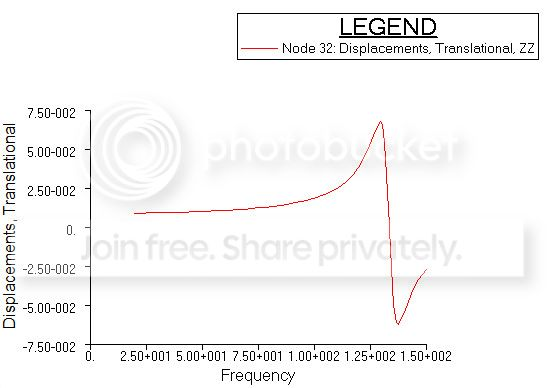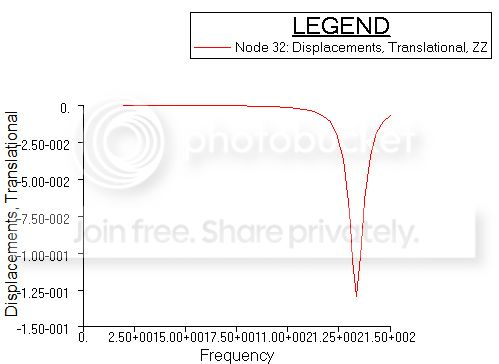# Structural FEM analysis: transiet response vs frequency response

I am running 2 simulations on a cantilever plate in Nastran: one is a transient analysis (time domain) and the other one is a frequency response analysis.

The transient analysis computes the response to time-varying excitations explicitly defined in the time domain. The equation it has to solve is

$M \ddot{u}(t) + C \dot{u}(t) + K u(t) = P(t)$

where $M,C,K$ are matrices and $u,P$ are vectors. The equations is solved by numerical integration (central difference method). $P(t)$ is a sinusoid at frequency equal to the $1^{st}$ natural frequency of the plate.

The frequency response compute the response to oscilatory excitation explicitly defined in the frequency domain. The computed responses are given as magnitude/phase (with respect to the forcing) or as real/imaginary components. The equation is

$[-\omega^2 M +i \omega C+K] u(\omega) = P(\omega)$

The forcing function is defined in the range $20-150 \ Hz$ to excite the first mode only.

Running the 2 analysis I would expect to obtain the same results in terms of maximum displacement (since I am considering only the first mode in both cases), but it is not so. The amplitudes in the time plot and in the magnitude plot do not match at all. Where the problem could be? Am I missing something?But if I plot the real/imaginary components, the amplitude of the real part (picture on the left) matches the time domain amplitude. How should I interpreted it?If $P(t)=\sin(\omega_1 t)$ where $\omega_1$ is the first eigenfrequency, then $P(\omega)$ is really only nonzero for that particular frequency, $\omega=\omega_1$. There is no need to consider any other frequency in the frequency domain.
• But this in the case I transform $P(t)$ via FFT to obtain $P(\omega)$. But in Nastran I have to define a load in the frequency domain: I set a frequency range and it consider a sine wave sweeping that frequency range – Rhei Jan 6 '15 at 7:27